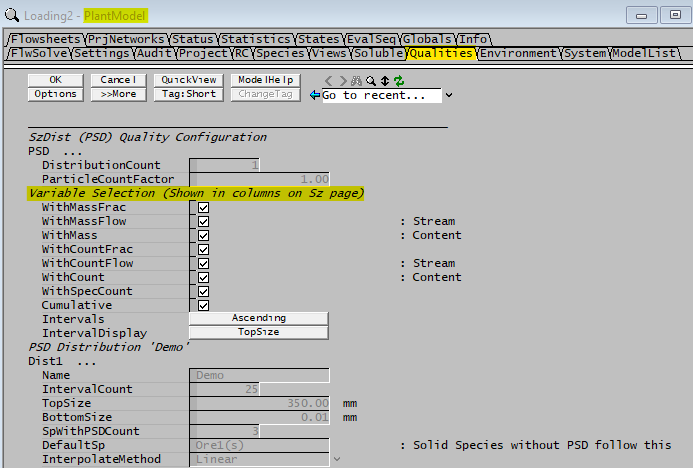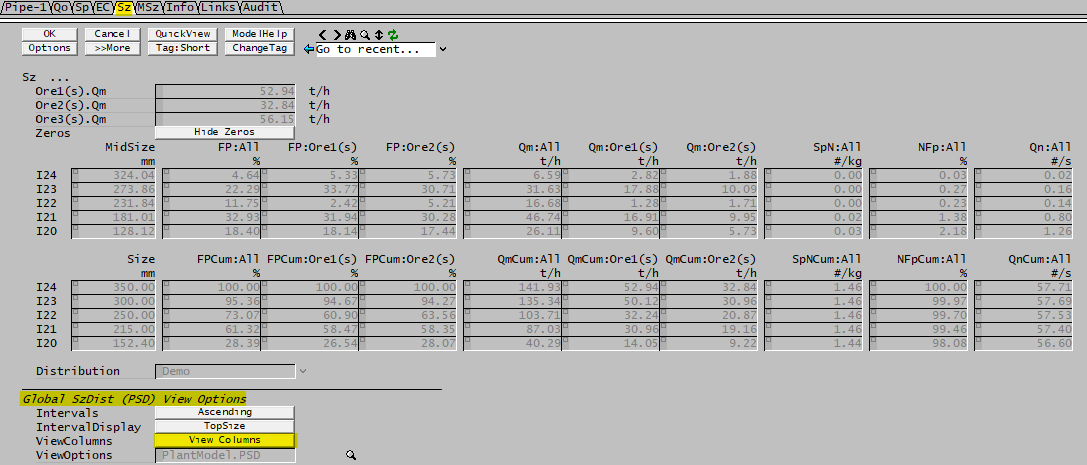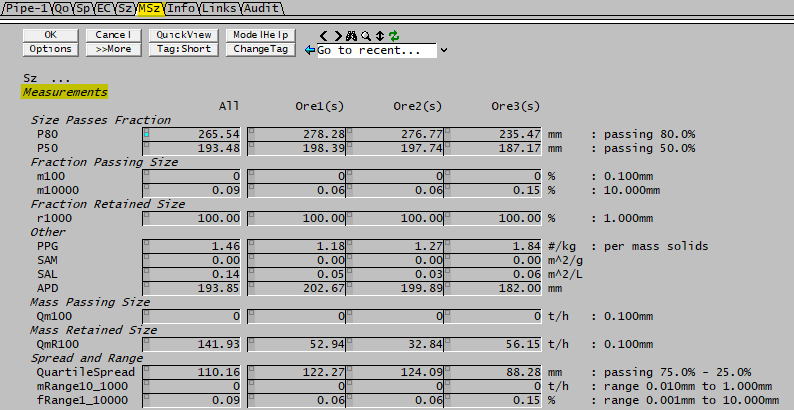# Size Distribution (PSD)

Navigation: Models ➔ Qualities Models ➔ Size Distribution (PSD)

## General Description

This option is used to define and use size distribution data in a project. This information can be used in Size Distribution Models such as the Crusher2 and Screen 2. This option is only be available to users with the Size Distribution add-on.

## Configuring Size Distribution

In order for the Size Distribution quality to be available in a project, it must included in the configuration file of the project.

Insert into Configuration file

Sort either by DLL or Group.

 DLL: SizeDst1.dll → Species Qualities → Size Distribution or Group: Size Distribution → Species Qualities → Size Distribution

The Size Distribution quality requires further configuration, including defining of a sieve series in the cfg file. Please refer to Size Configuration for more information.

## Creating a Size Distribution in a Stream

Open a project which uses a configuration file with Size Distribution configured (as described above).

Creating a Size Distribution in a feeder or pipe:

• On the Feeder|Content OR Pipe|Qi tab page, under Qualities, click on the SzDist.Action list box and select 'Create' (see Qualities Models - Fields and Actions for more information).
• New tab pages called 'DSz', 'Sz' and 'MSz' will become visible.
• The user can define the Size Distribution on the 'DSz' page as described in Size Distribution Definition.

## Modify or Remove a Size Distribution

Once a Size Distribution has been created, it will perpetuate through all subsequent units. The Size Distribution can be modified or the Size Distribution quality can be removed altogether in the same way as other Qualities. Please refer to Qualities Models - Fields and Actions for more information.

Note: You may add size distribution back into the plant at any downstream point - this may be the same size distribution, or it can be a different distribution. For example, you may use a coarse size distribution at the front end of the plant for crushing and milling ore and then have a fine size distribution at the back end of the plant for product handling.

## Changing Size Distributions

If the Size Distribution needs to be changed on the Feeder level (where the size distribution is created), then the user can simply select the size distribution to be used on the Sz tab page and redefine the size distribution. However, if the size distribution changes down stream - eg, it changes from the coarse distribution to the fine distribution, then the stream of material must pass through the Sieve Changing module unit operation to convert the size distribution.

NOTE: This also applies to mixing of streams with different size distributions. They must be converted to the same distribution before mixing; otherwise, incorrect sizes will result.

## Size Data Sections (Sz Tab)

Sz Tab Page

The variable display selection can be altered globally from the menu command View | Plant Model - Qualities Tab page.The view below shows the Sz tab of a pipe access window with all fields displayed.• You may select to show / hide a number of display fields using the View Columns button at the bottom of the pipe access window shown below. To Show or Hide any view, just click on that view from the list.
• The order of the size intervals may be changed by pressing the Intervals button to make it either ascending or descending.
• The Interval display can be shown as Topsize or Midsize for the size interval.

This Tab page displays the parameters described in the next section for each solid with a specified size distribution.

1. If you have 2 (or more) solids, Ore1 and Ore2 (as shown in the image above), and each has been specified with a size distribution, then you can choose to see values for each of these ores.
2. If there are any solids that were NOT specified to have an associated size distribution in Configuration file, then these solids will be combined with the Default solid (specified in the Configuration file) and SysCAD will assign the same size distribution to them as to the Default solid.
3. The mass flow shown for the Default solid will include the flow of the default solid as well as all solids that were NOT specified to have an associated size distribution in Size Configuration file.

### Sz Parameter Definitions

Parameter Functions / Descriptions Notes
MidSize / TopSize:

SysCAD calculates the MidSize for each size interval using the Geometric Mean (See notes below):

Depends on the Interval Display selection at the bottom of the tab page.
FP: Fraction passing of the solids - this is the fraction of solids in a size interval. Select WithMassFrac from Plant Model - Qualities
FPCum: Cumulative Fraction passing of the solids, summing from the smallest size fraction to the largest. Select WithMassFrac and Cumulative from Plant Model - Qualities
Qm: Mass flowrate of the solid within the size interval Select WithMassFlow from Plant Model - Qualities
QmCum: Cumulative Mass flowrate of the solids, summing from the smallest size fraction to the largest. Select WithMassFlow and Cumulative from Plant Model - Qualities
M: Mass of the solid within the size interval Select WithMass from Plant Model - Qualities (dynamic contents only)
MCum: Cumulative Mass of the solids, summing from the smallest size fraction to the largest. Select WithMass and Cumulative from Plant Model - Qualities (dynamic contents only)
SpNCum: Cumulative Number of Particles per unit mass of solids, summing from the smallest size fraction to the largest. Select WithSpecCount and Cumulative from Plant Model - Qualities
NFp: Number of Particles for the size interval as a percentage of the total number of particles. Calculation shown below:
• $\mathbf{\mathit{NF_{Pi}=\cfrac{Qn_i}{\sum Qn_i}\quad OR\quad NF_{Pi}=\cfrac{S_PN_i}{\sum S_PN_i}}}$
Select WithCountFrac from Plant Model - Qualities
NFpCum: Cumulative Number of Particles for the size interval as a percentage of the total number of particles, summing from the smallest size fraction to the largest. Select WithCountFrac and Cumulative from Plant Model - Qualities
Qn: Number of Particles per unit time. To calculate Qn for a Size Interval where the interval is i :
• $\mathbf{\mathit{Qn_i=\cfrac{mass solids_i}{Mean Particle Mass_i}\times CountFactor}}$
See notes below for Mean Particle Mass equation.
Select WithCountFlow from Plant Model - Qualities
QnCum: Cumulative Number of Particles per unit time, summing from the smallest size fraction to the largest. Select WithCountFlow and Cumulative from Plant Model - Qualities
N: Number of Particles within the size interval. To calculate Qn for a Size Interval where the interval is i :
• $\mathbf{\mathit{Qn_i=\cfrac{mass solids_i}{Mean Particle Mass_i}\times CountFactor}}$
See notes below for Mean Particle Mass equation.
Select WithCount from Plant Model - Qualities
NCum: Cumulative Number of Particles within the size interval, summing from the smallest size fraction to the largest. Select WithCount and Cumulative from Plant Model - Qualities
SpN:

Number of Particles per unit mass of solids. To calculate the SpN for a Size Interval where the interval is i :

• $\mathbf{\mathit{SpN_i=\cfrac{\cfrac{mass solids_i}{Mean Particle Mass_i}\times CountFactor}{Total Mass of Solids}}}$
See notes below for Mean Particle Mass equation.
Select WithSpecCount from Plant Model - Qualities

NOTES:

1. Geometric Mean - The geometric mean is not always displayed, but is used in most Size Distribution Models
SysCAD also calculates the Geometric mean for each size fraction as shown below:
• $Geometric Mean_i = \sqrt{d_i * d_{i-1}}$
except in the case of i = 0, where the following equation is used:
• $Geometric Mean_0 = \sqrt{d_0 * d_{bottom}}$
where dbottom is the Bottom Size defined by the user in the Size Distribution section of the Size Configuration.
2. Mean Particle Mass: based on Geometric Mean given above.
• $\mathbf{\mathit{Mean Particle Mass_i=\cfrac{\pi}{6} * Geometric Mean_i^3 \, * \rho_{species}}}$
3. CountFactor- The CountFactor mentioned in the SpN and Qn equations is specified in the Measurements Tab of the Model configuration.
• This is a correction which is applied when calculating particle numbers from masses. It allows client specific models which have different characteristic average particle sizes in a bin to correctly display particles numbers. Further if PSD calculations implemented in client specific model are based on numbers (as is the case for agglomeration for example), the count correction is applied when these numbers are calculated, and when the numbers are reconverted to mass fractions.
• SysCAD uses the Geometric Mean as described above for "average" diameter of a size interval. However there are other ways of characterizing the 'typical' diameter of a particle in a size interval (bin). For example, if the diameters are uniformly distributed between the minimum and maximum diameter of a particular bin, the volumes will not be uniformly distributed.
• To illustrate, suppose we have a bin containing particles with diameters between 1 and 2 microns. The volumes of these particles will range between $\mathbf{\mathit{\pi/6}}$ and $\mathbf{\mathit{8\pi/6}}$ cubic microns.
Using an arithmetic mean the average volume would be $\mathbf{\mathit{3.375\pi/6}}$, whereas for this particular distribution, the average volume is $\mathbf{\mathit{3.75\pi/6}}$, corresponding to a particle of diameter $\mathbf{\mathit{3.75^{1/3}=1.55361}}$ microns.
The difference factor is $\mathbf{\mathit{1.55361/1.5 = 1.035744}}$

## Size Measurements Data Sections (MSz Tab)

By default, the MSz Tab Page the variables displayed on this tab page are either by default or user defined on the configuration file Measurements tab. See Measurements for further information and configuration of measurements.

For example, some display fields that may be present are shown below:### MSz Parameter Definitions

 Size Passes Fraction P80: Size passing fraction = 80%. Fraction set in the configuration file. P50: Size passing fraction = 50%. Fraction set in the configuration file. Fraction Passing Size m100: Fraction passing 100 microns. The size interval definition is set in the configuration file. m10000: Fraction passing 10000 microns (10 mm). The size interval definition is set in the configuration file. Fraction Retained Size r1000: Cumulative retained fraction at specified size of 1000 microns (1mm). The size interval definition is set in the configuration file. Other PPG: Total Particle Count per Mass. This is calculated as shown below: $PPG = \cfrac{\sum {\cfrac{SpeciesMass_i}{Mean Particle Mass_i}}}{TotalSolidMass}$ Note: Mean Particle Mass is based on Geometric Mean Note: This value ignores the user defined Count Factor SAM: Surface Area Mass basis (per Gram). This is calculated as shown below: $SAM = \cfrac{\sum AG_i}{TotalSolidMass}$ where $AG_i = 0.001 * \cfrac{3 * Species Particle Mass_i}{Species Density * 0.5 * Geometric Mean Diameter_i}$ i is for each size interval for each solid species SAL: Surface Area per Liter $SAL = \cfrac{SAM * TotalSolidMass}{TotalLiquidVolume}$ where TotalLiquidVolume is at mixture temperature NOTE: Interpolation method used for measurements is selected by the user in Size Distribution Configuration and is usually on the cumulative distribution. SAM and SAL are calculated based on ALL solids species, unlike the calculation for SAM and SAL in SSA Quality where only the one nominated solids species is used. The Geometric Mean is used for calculating "average" diameter of size interval. APD Average particle diameter based on Fraction Passing and Geometric mean size of each size interval Mass Passing Size Qm100 Cumulative passing mass at specified size. The size interval definition is set in the configuration file. Mass Retained Size QmR100 Cumulative retained mass at specified size. The size interval definition is set in the configuration file. Spread and Range QuartileSpread Difference in sizes at two specified cumulative passing fractions:75.0% - 25.0%. Range definition is set in the configuration file. mRange10_1000 Mass flow between two different size ranges: 0.010mm to 1.000mm. Range definition is set in the configuration file. fRange1_10000 Mass Fraction between two different size ranges: 0.001mm to 10.000mm. Range definition is set in the configuration file.